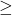Question

negative six times a number is at least 14

1.-6x14

Step-by-step explanation:

For this inequality let x stand for a number. In algebra, when multiplying a constant and a variable, just put the constant next to the variable with no sign in between. In this inequality, -6 is the constant and x is the variable, so the left side of the inequality should be -6x. Then it says this monomial is at least 14, this means it is greater than or equal to, so the sign in between both sides should be. Therefore, the final answer is –6x14.

2.Latifah